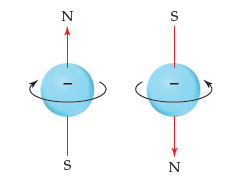# Problem: Electron spin. The electron behaves as if it were spinning about an axis, thereby generating a magnetic field whose direction depends on the direction of spin. The two directions for the magnetic field correspond to the two possible values for the spin quantum number, ms.From this figure, why are there only two possible values for the spin quantum number?

###### FREE Expert Solution

Based on the given figure, we're asked to explain why are there only two possible values for the spin quantum number, ms.

Recall that the spin quantum number determines the direction of the electron spin.

From the figure, we can see that there are only two possible directions of the spin for each magnetic field:

100% (443 ratings)###### Problem DetailsElectron spin. The electron behaves as if it were spinning about an axis, thereby generating a magnetic field whose direction depends on the direction of spin. The two directions for the magnetic field correspond to the two possible values for the spin quantum number, ms.

From this figure, why are there only two possible values for the spin quantum number?